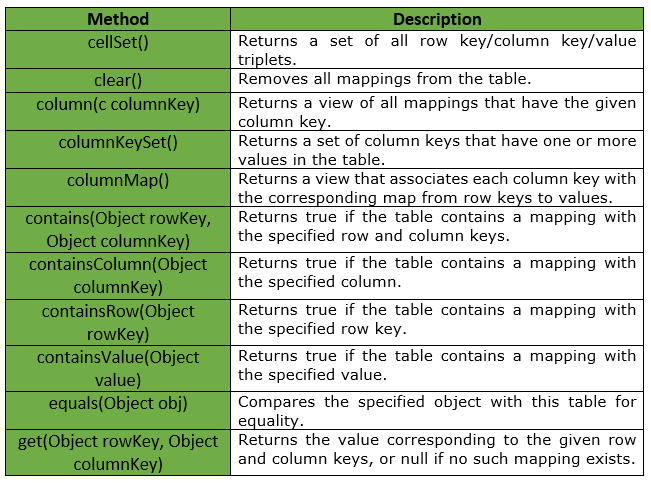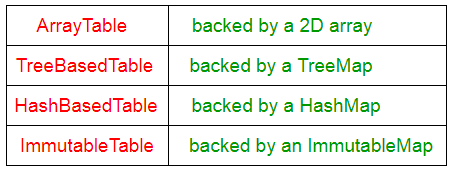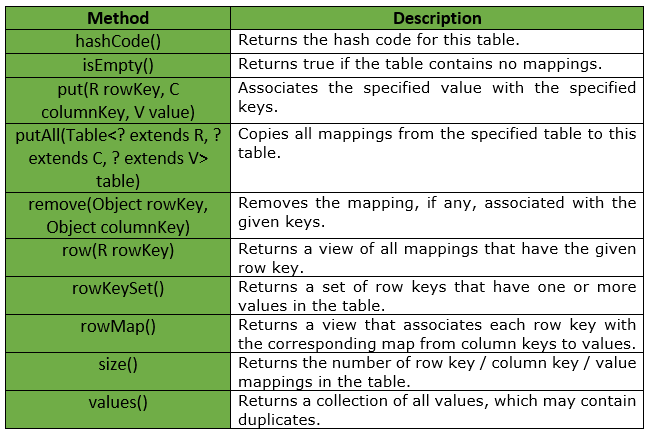GeeksforGeeks App
Open AppBrowser
Continue

# Table | Guava | Java

Guava’s Table is a collection that represents a table like structure containing rows, columns and the associated cell values. The row and the column act as an ordered pair of keys.

The row and column act as an ordered pair of keys. If we have to manage this the traditional way then the structure would be a map of rows where each row contain a map of column and the cell values, for example, Map<rowKey, Map<colKey, cellValue>>.

Declaration : Following is the declaration for com.google.common.collect.Table<R, C, V> interface :

```@GwtCompatible
public interface Table<R, C, V>
```

Parameters :

• R : The type of the table row keys.
• C : The type of the table column keys.
• V : The type of the mapped values.

Some of methods provided by Table Interface are :Guava provides different variety of implementations for Table interface, which are shown in below table :Important Points :

• A table may be sparse, with only a small fraction of row key / column key pairs possessing a corresponding value.
• In some implementations, data access by column key may have fewer supported operations or worse performance than data access by row key.
• All methods that modify the table are optional, and the views returned by the table may or may not be modifiable. When modification isn’t supported, those methods will throw an UnsupportedOperationException.

Below given are some other methods provided by Table Interface :Example :

 `// Java code to show implementation of``// Guava's Table interface``import` `java.util.Map;``import` `java.util.Set;`` ` `import` `com.google.common.collect.HashBasedTable;``import` `com.google.common.collect.Table;`` ` `class` `GFG {`` ` `    ``// Driver method``    ``public` `static` `void` `main(String args[])``    ``{`` ` `        ``// creating a table to store Student information``        ``Table studentTable = HashBasedTable.create();`` ` `        ``// Adding student details in the table``        ``// The first field represents the department``        ``// of student, second field represents the``        ``// Roll no. and third field represents the``        ``// student name``        ``studentTable.put(``"CSE"``, ``"5"``, ``"Dhiman"``);``        ``studentTable.put(``"CSE"``, ``"7"``, ``"Shubham"``);``        ``studentTable.put(``"CSE"``, ``"9"``, ``"Abhishek"``);``        ``studentTable.put(``"CSE"``, ``"12"``, ``"Sahil"``);`` ` `        ``studentTable.put(``"ECE"``, ``"15"``, ``"Ram"``);``        ``studentTable.put(``"ECE"``, ``"18"``, ``"Anmol"``);``        ``studentTable.put(``"ECE"``, ``"20"``, ``"Akhil"``);``        ``studentTable.put(``"ECE"``, ``"25"``, ``"Amrit"``);`` ` `        ``// get Map corresponding to ECE department``        ``Map eceMap = studentTable.row(``"ECE"``);`` ` `        ``System.out.println(``"List of ECE students : "``);`` ` `        ``for` `(Map.Entry student : eceMap.entrySet()) {``            ``System.out.println(``"Student Roll No : "` `+ student.getKey() + ``", Student Name : "` `+ student.getValue());``        ``}`` ` `        ``System.out.println();`` ` `        ``// get a Map corresponding to Roll no. 12``        ``Map stuMap = studentTable.column(``"12"``);`` ` `        ``for` `(Map.Entry student : stuMap.entrySet()) {``            ``System.out.println(``"Student Roll No : "` `+ student.getKey() + ``", Student Name : "` `+ student.getValue());``        ``}``    ``}``}`

Output :

```List of ECE students :
Student Roll No : 15, Student Name : Ram
Student Roll No : 18, Student Name : Anmol
Student Roll No : 20, Student Name : Akhil
Student Roll No : 25, Student Name : Amrit

Student Roll No : CSE, Student Name : Sahil
```